Multivariable Calculus : Tangents & Normals

Example Questions

Example Question #1 : Multivariable Calculus

Find the equation of the tangent plane to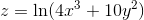at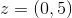.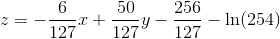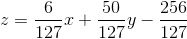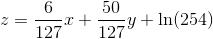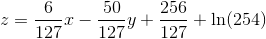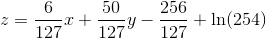Explanation:

First, we need to find the partial derivatives in respect to, and, and plug in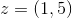.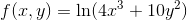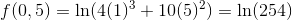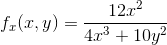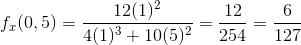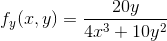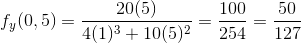Remember that the general equation for a tangent plane is as follows: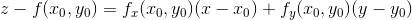Now lets apply this to our problem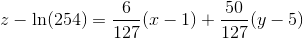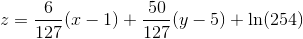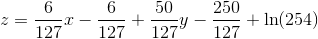Example Question #2 : Multivariable Calculus

Find the equation of the tangent plane toat.Explanation:

First, we need to find the partial derivatives in respect to, and, and plug in.Remember that the general equation for a tangent plane is as follows:Now lets apply this to our problem